Download Presentation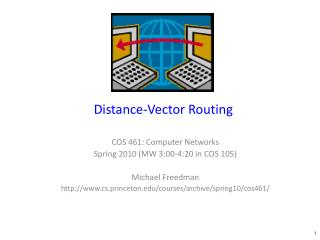Distance-Vector Routing

# Distance-Vector Routing - PowerPoint PPT Presentation

Distance-Vector Routing. COS 461: Computer Networks Spring 2010 (MW 3:00-4:20 in COS 105) Michael Freedman http://www.cs.princeton.edu/courses/archive/spring10/cos461/. Shortest-Path Routing. 2. 1. 3. 1. 4. 2. 1. 5. 4. 3. Path-selection model Destination-basedI am the owner, or an agent authorized to act on behalf of the owner, of the copyrighted work described.
Download Presentation## Distance-Vector Routing

An Image/Link below is provided (as is) to download presentation

Download Policy: Content on the Website is provided to you AS IS for your information and personal use and may not be sold / licensed / shared on other websites without getting consent from its author.While downloading, if for some reason you are not able to download a presentation, the publisher may have deleted the file from their server.

- - - - - - - - - - - - - - - - - - - - - - - - - - E N D - - - - - - - - - - - - - - - - - - - - - - - - - -
Presentation Transcript### Distance-Vector Routing

COS 461: Computer Networks

Spring 2010 (MW 3:00-4:20 in COS 105)

Michael Freedman

http://www.cs.princeton.edu/courses/archive/spring10/cos461/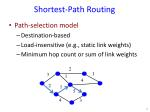Shortest-Path Routing

2

1

3

1

4

2

1

5

4

3

• Path-selection model
• Destination-based
• Minimum hop count or sum of link weights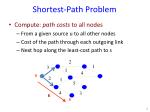Shortest-Path Problem

2

1

3

1

4

u

2

1

5

4

3

6

s

• Compute: path costs to all nodes
• From a given source u to all other nodes
• Cost of the path through each outgoing link
• Next hop along the least-cost path to s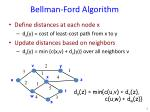Bellman-Ford Algorithm

2

v

y

1

3

1

4

x

z

u

2

1

5

t

du(z) = min{c(u,v) + dv(z),

c(u,w) + dw(z)}

w

4

3

s

• Define distances at each node x
• dx(y) = cost of least-cost path from x to y
• Update distances based on neighbors
• dx(y) = min {c(x,v) + dv(y)} over all neighbors v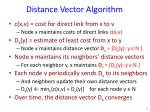Distance Vector Algorithm
• c(x,v) = cost for direct link from x to v
• Node x maintains costs of direct links c(x,v)
• Dx(y) = estimate of least cost from x to y
• Node x maintains distance vector Dx = [Dx(y): y є N ]
• Node x maintains its neighbors’ distance vectors
• For each neighbor v, x maintains Dv = [Dv(y): y є N ]
• Each node v periodically sends Dv to its neighbors
• And neighbors update their own distance vectors
• Dx(y) ← minv{c(x,v) + Dv(y)} for each node y ∊ N
• Over time, the distance vector Dx converges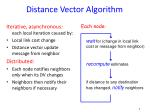Iterative, asynchronous: each local iteration caused by:

Local link cost change

Distance vector update message from neighbor

Distributed:

Each node notifies neighbors only when its DV changes

Neighbors then notify their neighbors if necessary

Distance Vector Algorithm

waitfor (change in local link cost or message from neighbor)

recompute estimates

if distance to any destination has changed, notifyneighbors

Each node: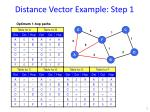Distance Vector Example: Step 1

Optimum 1-hop paths

E

C

3

1

F

1

2

6

1

D

3

A

4

B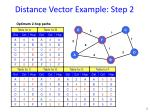Distance Vector Example: Step 2

Optimum 2-hop paths

E

C

3

1

F

1

2

6

1

D

3

A

4

B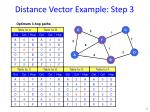Distance Vector Example: Step 3

Optimum 3-hop paths

E

C

3

1

F

1

2

6

1

D

3

A

4

B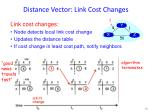Distance Vector: Link Cost Changes

1

4

1

50

X

Z

Y

• Link cost changes:
• Node detects local link cost change
• Updates the distance table
• If cost change in least cost path, notify neighbors

algorithm

terminates

“good

news

travels

fast”Distance Vector: Link Cost Changes

60

4

1

50

X

Z

Y

• Link cost changes:
• Good news travels fast
• Bad news travels slow - “count to infinity” problem!

algorithm

continues

on!Distance Vector: Poison Reverse

60

4

1

50

X

Z

Y

• If Z routes through Y to get to X :
• Z tells Y its (Z’s) distance to X is infinite (so Y won’t route to X via Z)
• Still, can have problems when more than 2 routers are involved

algorithm

terminatesRouting Information Protocol (RIP)
• Distance vector protocol
• Nodes send distance vectors every 30 seconds
• … or, when an update causes a change in routing
• Link costs in RIP
• All links have cost 1
• Valid distances of 1 through 15
• … with 16 representing infinity
• Small “infinity”  smaller “counting to infinity” problem
• RIP is limited to fairly small networks
• E.g., used in the Princeton campus networkComparison of LS and DV Routing

Message complexity

• LS: with n nodes, E links, O(nE) messages sent
• DV: exchange between neighbors only

Speed of Convergence

• LS: relatively fast
• DV: convergence time varies
• May be routing loops
• Count-to-infinity problem

Robustness: what happens if router malfunctions?

LS:

• Node can advertise incorrect link cost
• Each node computes only its own table

DV:

• DV node can advertise incorrect path cost
• Each node’s table used by others (error propagates)Similarities of LS and DV Routing
• Shortest-path routing
• Metric-based, using link weights
• Routers share a common view of how good a path is
• As such, commonly used inside an organization
• RIP and OSPF are mostly used as intradomain protocols
• E.g., Princeton uses RIP, and AT&T uses OSPF
• But the Internet is a “network of networks”
• How to stitch the many networks together?
• When networks may not have common goals
• … and may not want to share information